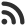Feed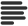Articles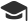Tutorials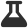Lab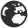CompaniesLeaderboard
 DG Student at BIT Mesra Oct. 12, 2020, 1:44 p.m. ⋅ 746 views

# TCS NQT Technical Questions set 2

31. What is the best case and worst case complexity of binary search ?

A. O(log n), O(n)
B. O(log n), O(log n)
C. O(1), O(1)
D. O(log n), O(1)

Ans. D

32. Using calloc() function, data members are initialized as :

A. garabage value
B. 0
C. 1
D. -1

Ans. B

33. How many kinds of classes are there in c++?

A. 1
B. 2
C. 3
D. 4

Ans. B

34. #include is called :

A. preprocessor directive
B. Inclusion directive
C. File Inclusion directive
D. None of these

Ans. A

35. Which of the following is not present in string.h ?

A. strcpy()
B. strapp()
C. strlen()
D. strcat()

Ans. B

36. C Programming Language is often called as :

A. High Level Language
B. Middle Level Language
C. Low Level Language
D. None of these

Ans. B

37. Which of the following is true about C programming language ?

A. C is a structural lang.
B. C is a procedural lang.
C. C does not supports function within function
D. All of these

Ans. D

38. How many keywords are there in C Programming Language ?

A. 28
B. 32
C. 21
D. None of these

Ans. B

39. Which is not the extended keyword in C ?

A. _Imaginary
B. _Complex
C. inline
D. None of these

Ans. D

40. If a function’s return type is not explicitly defined then it’s default to

A. int
B. char
C. float
D. void

Ans. A

41. An object of type ‘char’ is of :

A. 1 byte
B. 2 bytes
C. 4 bytes
D. 8 bytes

Ans. A

42. For 32 bits environment, size of ‘int’ data type is :

A. 2 bytes
B. 4 bytes
C. 8 bytes
D. None of these

Ans. B

43. In C, the names of variables, functions, labels, and various other user-defined items are :

A. Keywords
B. Tokens
C. Identifiers
D. None of these

Ans. C

44. Which operator is used to return the length of the variables in bytes ?

A. size()
B. length()
C. leng()
D. sizeof()

Ans. D

45. Process in which source code is combined with object code is termed as :

D. None of these

Ans. C

46. _________ is used to break out of a program ?

A. break
B. continue
C. terminate
D. exit

Ans. D

47. Functions with same name and different parameters represents :

B. function overriding
C. recursion
D. None of the mentioned

Ans. A

48. Which precision specifier the number of significant digits ?

A. %g
B. %G
C. %f
D. Both a and b

Ans. D

49. In order to display address, we need :

A. %q
B. %a
C. %p
D. None of these

Ans. C

50. The format identifier ‘%i’ is also used for :

A. char
B. int
C. float
D. double

Ans. B

51. Can we declare function inside structure of C ?

A. Yes
B. No
C. Warning

Ans. B

52. Which of the following is an ternary operator in C?

A. \$\$
B. &&
C. !*
D. ?:

Ans. D

53. Null function is also known as ______

A. Anonymous Function
B. Generic Function
C. Void Function
D. Null Operator

Ans. D

54. A complete binary tree has a property that the value at each node is at least as large as the values at its children nodes. What is this binary tree known as?

A. BST
B. AVL Tree
C. Complete Balanced Tree
D. Heap

Ans. D

55. In pointers, meaning of ‘*’ is :

A. pointer variable
D. None of the above

Ans. C

56. A librarian has to rearrange the library books on a shelf in a proper order at the end of each day. Which of the following sorting techniques should be the librarian’s ideal choice?

A. Bubble Sort
B. Insertion Sort
C. Selection Sort
D. Heap Sort

Ans. B

57. Which of the following concept is for stack implementation ?

B. BFS
C. Recursion
D. None of these

Ans. C

58. What is the average time required to perform a successful sequential search for an element in an array A[1:n] ?

A. (n+1)/2
B. log n
C. n(n+1)/2
D. n^2

Ans. A

59. How are protected members of a base class accessed in the derived class when inherited privately in C++ ?

A. Privately
B. Publicly
C. Protectedly
D. Not inherited

Ans. A

60. Which of the following sorting algorithms yields approximately the same worst-case and average-case running time behavior in O(n log n)?

A. Bubble sort & Selection sort
B. Heap sort & Merge sort
C. Quick sort & Radix Sort
D. Tree Sort & Quick Sort

Ans. B

Events

Nov. 28, 2018, 5:30 p.m.

Python from zero to hero

place Delhi

Aug. 13, 2018, 5:30 p.m.

Python from zero to hero

place Bangalore ( HackersFriend office BTM Layout)